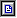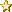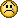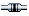This forum is now READ-ONLY! Please visit http://forum.wiring.co/ for the new forum.

Wiring ForumProgramming Questions & HelpSyntax › arduino but syntax problem and desperatly urgentPages: 1arduino but syntax problem and desperatly urgent (Read 5344 times)01/22/07 at 07:51:17
 llumen   Offline YaBB Newbies I Love YaBB 2! Posts: 1Hi I'm writing a thundermaschine, originally in max but it doesn't seem to handle the speed I need well so am trying to write everything in the arduino software, but since it's practically the same as processing I'm going to try my luck here as well (I have very little time to get this installation finished) Could someone take a look at the included script, and could tell someone if this is an approach that would work? I need to have 4 solenoids that oscillate (vibrate) or hit very large drums and am trying to get them coded straight on the arduino board.   int solenoid1;  int solenoid2;  int solenoid3;  int solenoid4;     int s1;  int s2;  int s3;  int s4;     // counters macro  int c1;  int c2;  int c3;  int c4;     //ontime macro  int on1;  int on2;  int on3;  int on4;     //offtime macro  int off1;  int off2;  int off3;  int off4;     //micro Osc  int cm1;  int cm2;  int cm3;  int cm4;     int sm1; //status micro    int sm2;  int sm3;  int sm4;     int oscOn1;  int oscOn2;  int oscOn3;  int oscOn4;     int oscOff1;  int oscOff2;  int oscOff3;  int oscOff4; void setup(){  // set pins  int solenoid1=13;  int solenoid2=12;  int solenoid3=11;  int solenoid4=10;     pinMode(solenoid1, OUTPUT);    pinMode(solenoid2, OUTPUT);    pinMode(solenoid3, OUTPUT);    pinMode(solenoid4, OUTPUT);          // status Solenoid (1=On 0=Off) macro  int s1=1;  int s2=0;  int s3=0;  int s4=0;     // counters macro  int c1=0;  int c2=0;  int c3=0;  int c4=0;     //ontime macro  int on1=5000;  int on2=180000;  int on3=180000;  int on4=180000;     //offtime macro  int off1=1000;  int off2=180000;  int off3=180000;  int off4=180000;     //micro Osc  int cm1=0;  int cm2=0;  int cm3=0;  int cm4=0;     int sm1=0; //status micro    int sm2=0;  int sm3=0;  int sm4=0;     int oscOn1=24;  int oscOn2=24;  int oscOn3=24;  int oscOn4=24;     int oscOff1=38;  int oscOff2=38;  int oscOff3=38;  int oscOff4=38;} void loop(){   c1 +=1;  if(s1=1){    if(c1=on1){ s1=0; c1=0;    }    micro1(); // call micro1 function  }  if(s1=0){    if(c1=off1){ s1=1; c1=0;    }  }  delay(1);} void micro1(){ // controls on off     if(sm1=1){    cm1 +=1;    if(cm1=oscOn1){ sm1=0; cm1=0;    }  digitalWrite(solenoid1,HIGH);  }  if(sm1=0){    cm1 +=1;    if(cm1=oscOff1){    sm1=1;    cm1=0;    }   digitalWrite(solenoid1,LOW);  }}IP LoggedReply #1 - 03/13/07 at 09:08:06
 MarkvdBos   Offline YaBB Newbies I love Electronic Media Art Delft, the Netherlands Posts: 2The first problem that I see is that you declare your variables twice.You should not use int solenoid1=13; in the setup() routine because then you will declare this variable local for the setup() routine.If you use solenoid1=13; (so without the int) you will use the solenoid1 variable declared global and you can refer to it in the loop() routine.Maybe this wil help.IP LoggedReply #2 - 03/18/07 at 02:17:24
 Alan_Kilian   Offline Full Member Wiring? It looks like Java to me. Posts: 118Can you describe what is not working?IP LoggedPages: 1